# 【MTSP】基于matlab遗传算法求解多旅行商问题【含Matlab源码 1338期】

## 二、遗传算法简介

1 引言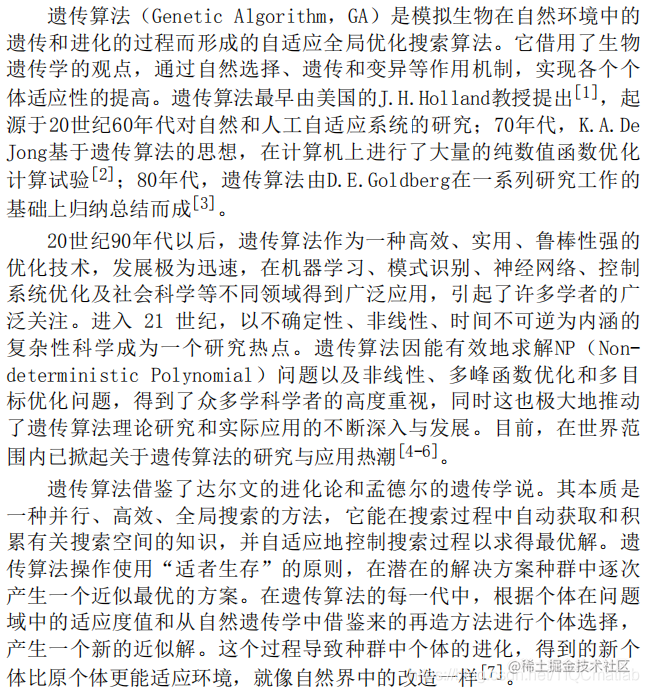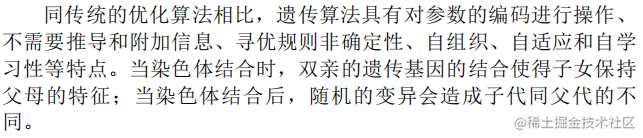2 遗传算法理论 2.1 遗传算法的生物学基础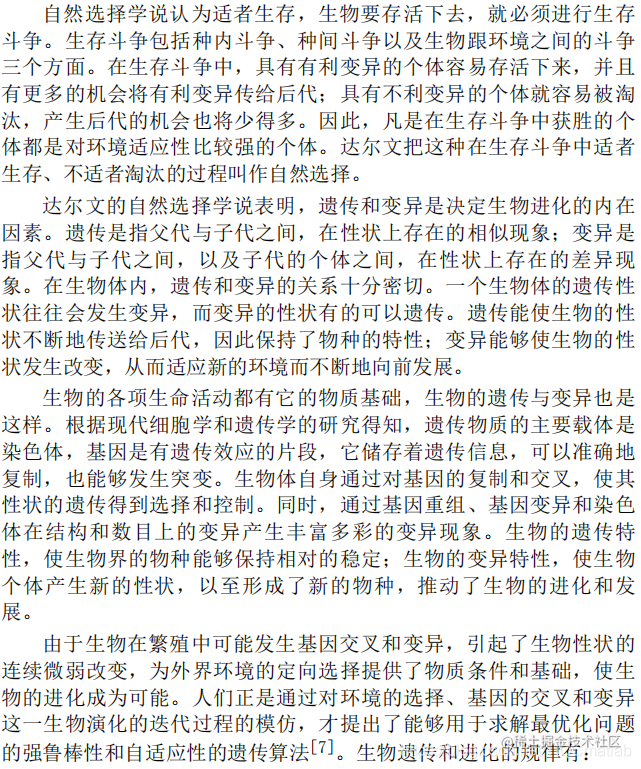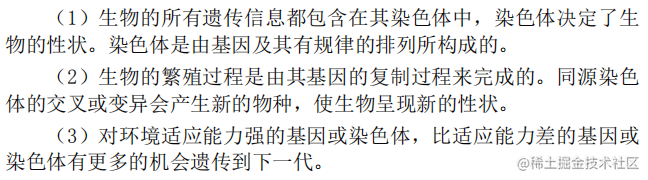2.2 遗传算法的理论基础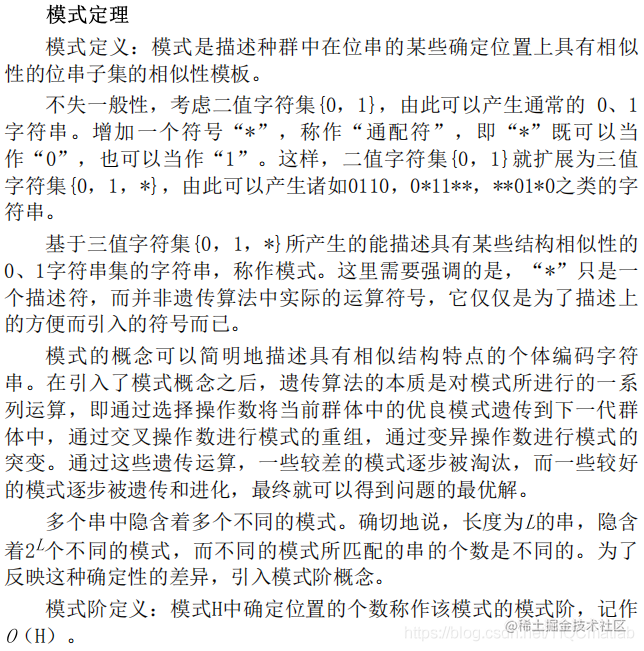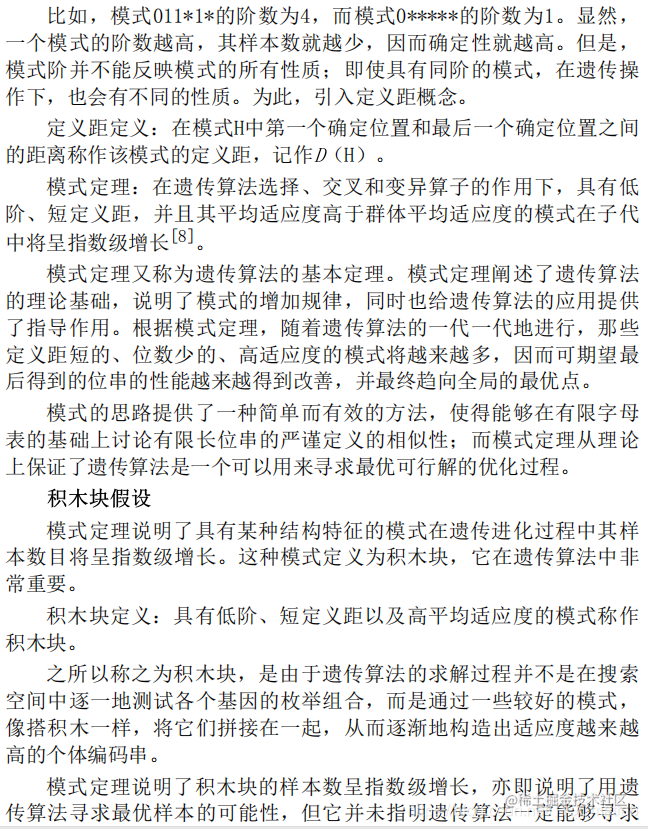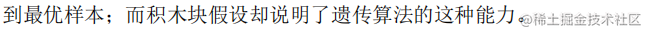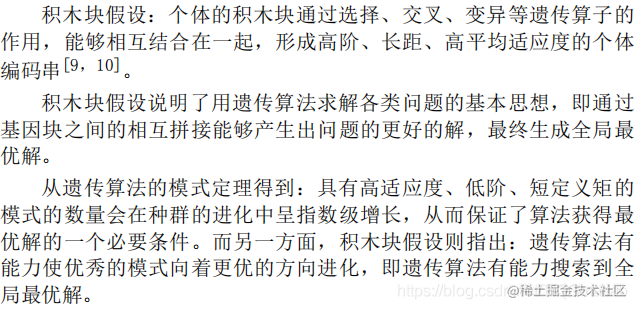2.3 遗传算法的基本概念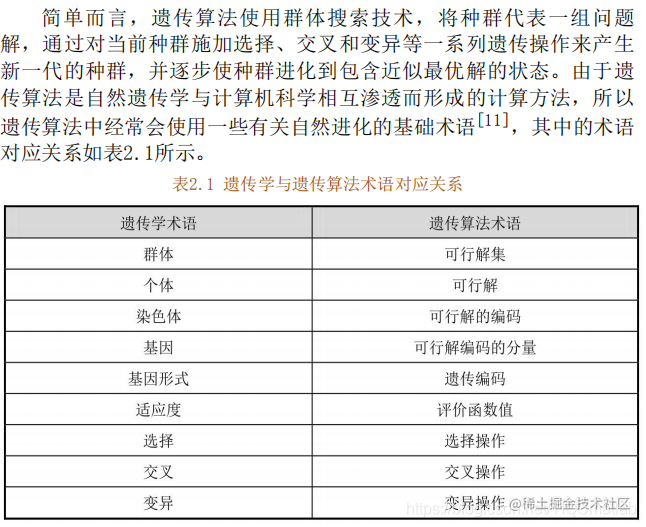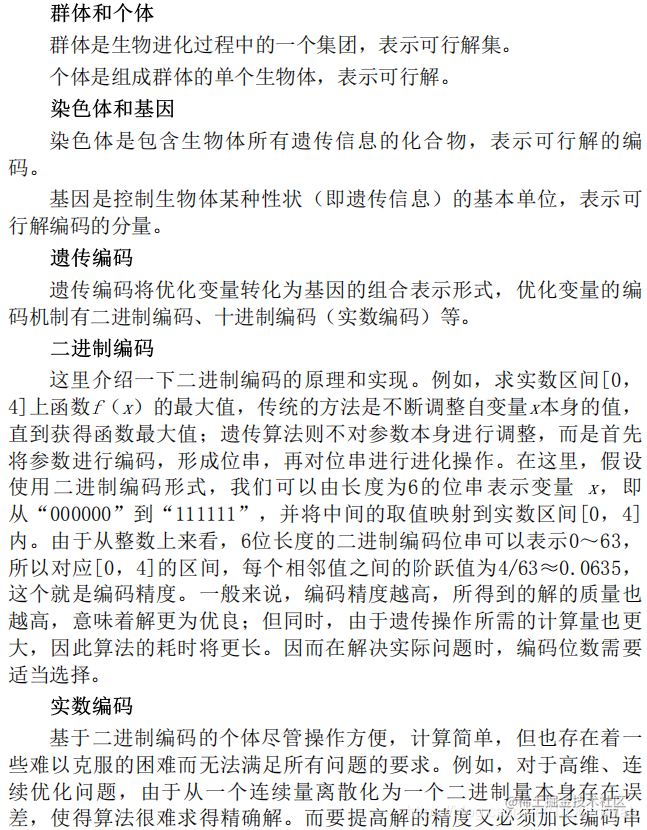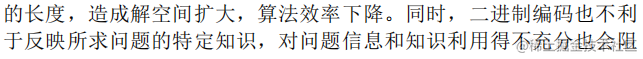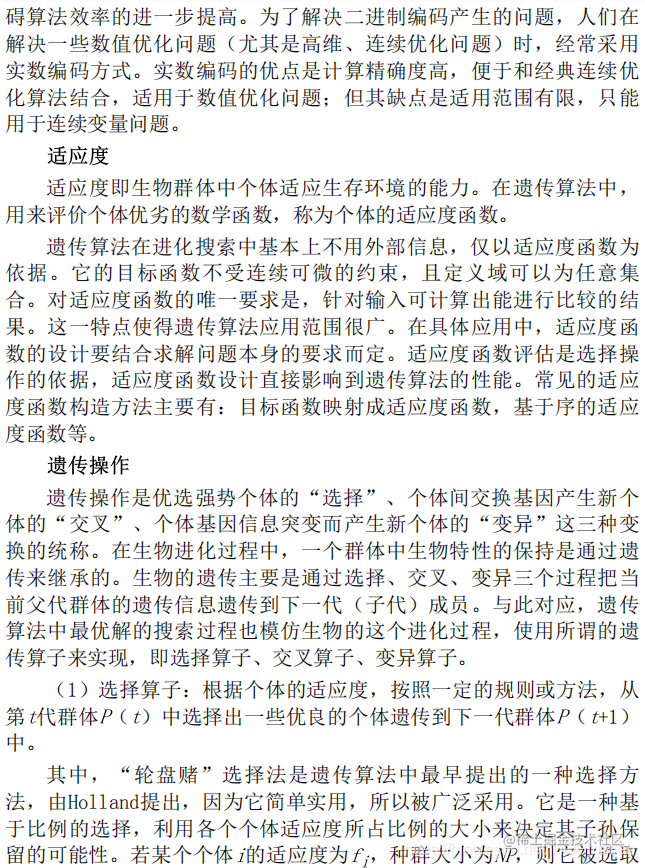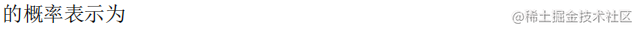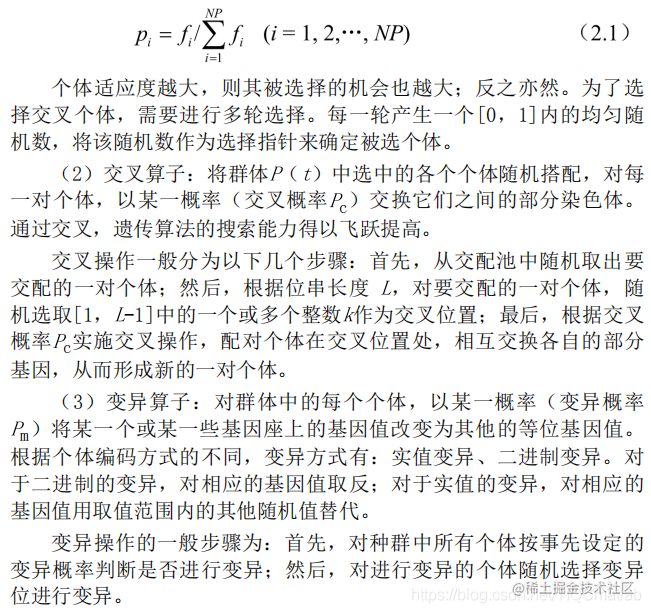2.4 标准的遗传算法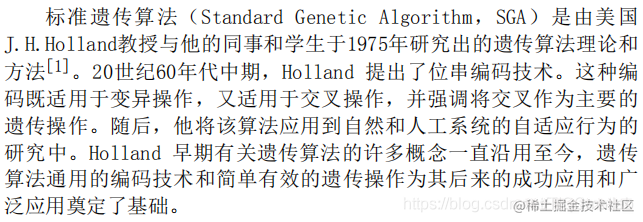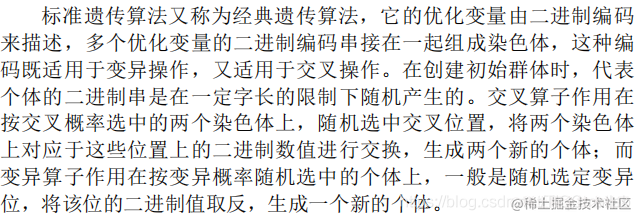2.5 遗传算法的特点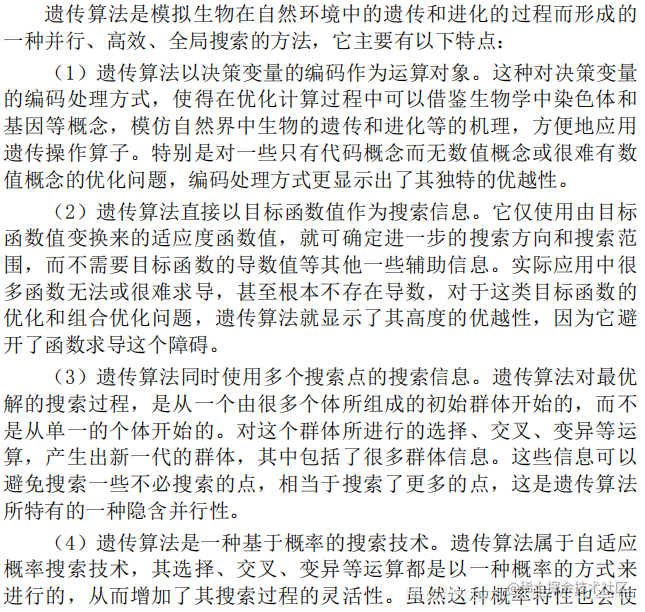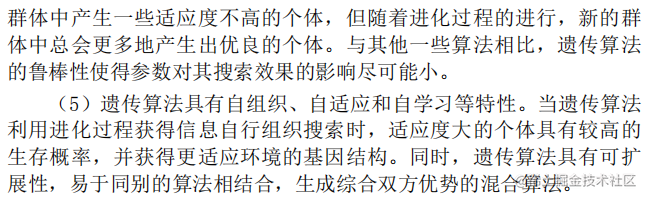2.6 遗传算法的改进方向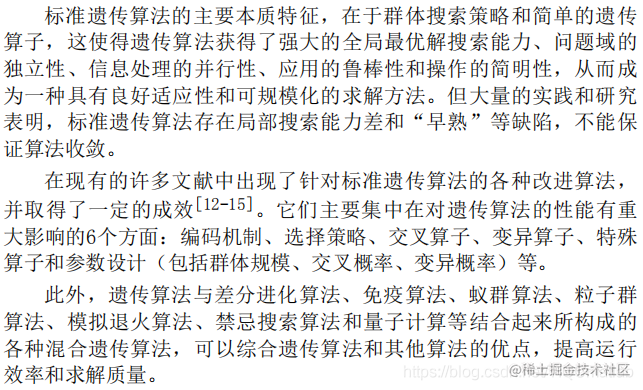3 遗传算法流程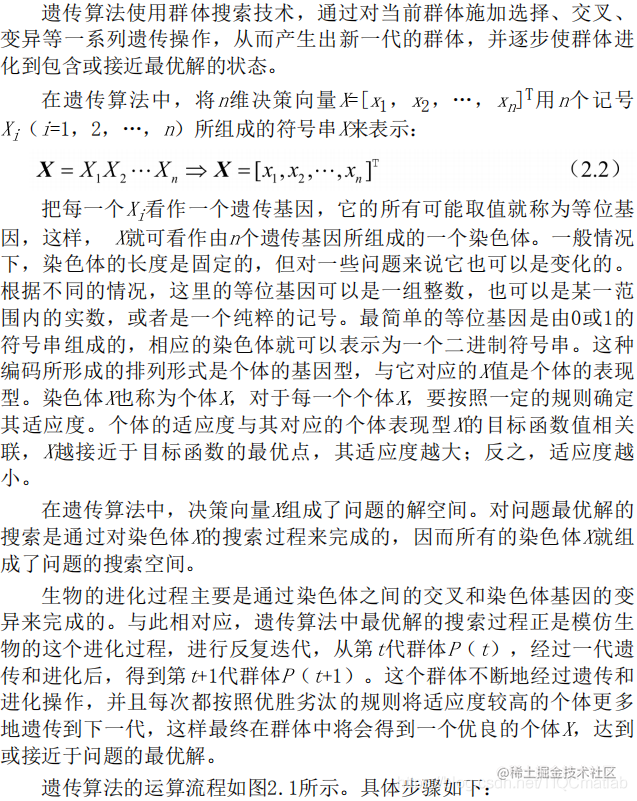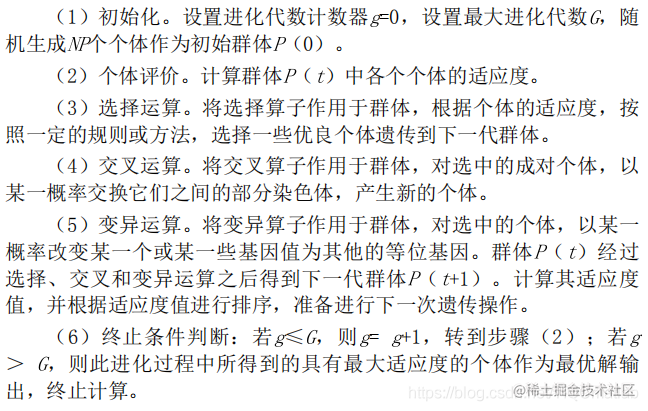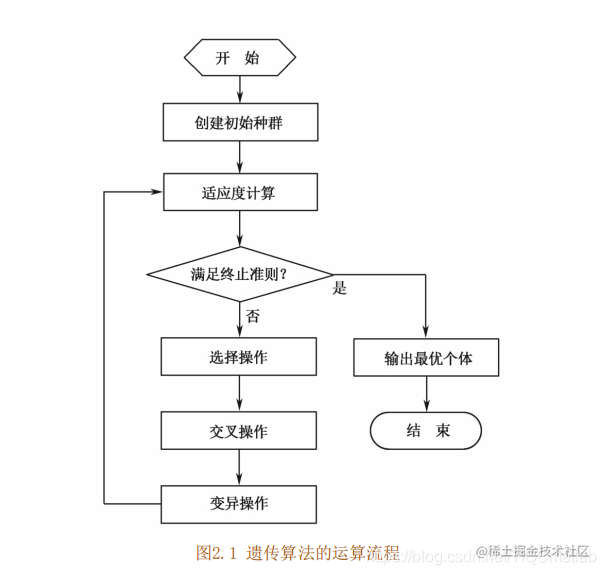4 关键参数说明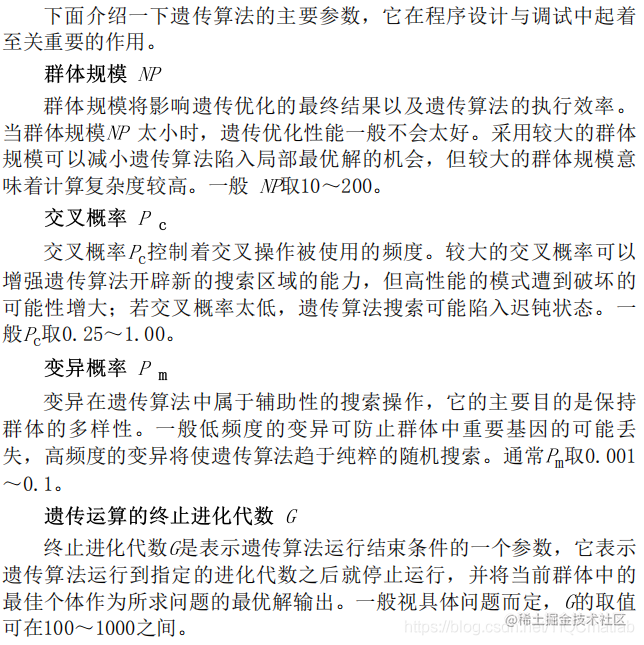## 三、部分源代码

``````function varargout = mtsp_ga_multi_ch(xy,dmat,salesmen,min_tour,max_tour,tw,pop_size,num_iter,use_complex,show_prog,show_res)
% MTSP_GA_MULTI_CH Multiple Traveling Salesmen Problem (M-TSP) Genetic Algorithm (GA) using multi-chromosome representation
%   Finds a (near) optimal solution to a variation of the M-TSP by setting
%   up a GA to search for the shortest route, taking into account
%   additional constraints, and minimizing the number of salesmen.
%
% Summary:
%     1. Each salesman starts at the first location, and ends at the first
%        location, but travels to a unique set of cities in between
%     2. Except for the first, each city is visited by exactly one salesman
%     3. The algorithm uses a special, so-called multi-chromosome genetic
%        representation to code solutions into individuals.
%     4. Special genetic operators (even complex ones) are used.
%     5. The number of salesmen used is minimized during the algorithm
%     6. Additional constraints have to be satisfied
%        - minimum number of locations, what each salesmen visit
%        - maximum distane travelled by each salesmen
%     7. Time windows can be defined for each locations (e.g. packing/loading times).
%
% Note: The Fixed Start/End location is taken to be the first XY point
%
% Inputs:
%     XY (float) is an Nx2 matrix of city locations, where N is the number of cities
%     DMAT (float) is an NxN matrix of city-to-city distances or costs
%     SALESMEN (scalar integer) is the number of salesmen to visit the cities
%     MIN_TOUR (scalar integer) is the minimum number of cities for each
%				salesmen, NOT including the start/end point
%     MAX_TOUR (scalar integer) is the maximum tour length for each salesmen
%     TW (scalar_integer) is the time window for each location
%     POP_SIZE (scalar integer) is the size of the population (should be divisible by 8)
%     NUM_ITER (scalar integer) is the number of desired iterations for the algorithm to run
%     USE_COMPLEX (scalar boolen 0/1) is the flag wether to use complex mutation operators or not
%     SHOW_PROG (scalar logical) shows the GA progress if true
%     SHOW_RES (scalar logical) shows the GA results if true
%
% Outputs:
%     OPT_RTE (integer array) is the best route found by the algorithm
%     MIN_DIST (scalar float) is the total distance traveled by the salesmen
%     OPT_ITER (scalar int) is the number of iterations until the optimal solution has been found
%	  OPT_TIME (scalar float) is the time in milliseconds until the optimal solution has been found
%     DIST_HISTORY (float array) is the history of distances of best found solutions
%
% Authors: Andras Kiraly, Janos Abonyi
% Email: kiralya@fmt.uni-pannon.hu
% Release Date: 16/10/2014
% The implementation is based on the work of Joseph Kirk: mtspf_ga
%
% *************************************************************************

% Process Inputs and Initialize Defaults
nargs = 11;
for k = nargin:nargs-1
switch k
case 0
xy = 40*rand(40,2);
case 1
N = size(xy,1);
a = meshgrid(1:N);
dmat = reshape(sqrt(sum((xy(a,:)-xy(a',:)).^2,2)),N,N);
case 2
salesmen = 8;
case 3
min_tour = 5;
case 4
max_tour = 100;
case 5
tw = 0;
case 6
pop_size = 80;
case 7
num_iter = 500;
case 8
use_complex = 0;
case 9
show_prog = 1;
case 10
show_res = 1;
otherwise
end
end

merging_prob = 0.3;

% Verify Inputs
[N,dims] = size(xy);
[nr,nc] = size(dmat);
if N ~= nr || N ~= nc
error('Invalid XY or DMAT inputs!')
end
n = N - 1; % Separate Start/End City

% Sanity Checks
salesmen = max(1,min(n,round(real(salesmen(1)))));
min_tour = max(1,min(floor(n/salesmen),round(real(min_tour(1)))));
pop_size = max(8,8*ceil(pop_size(1)/8));
num_iter = max(1,round(real(num_iter(1))));
show_prog = logical(show_prog(1));
show_res = logical(show_res(1));

% Initializations for Route Break Point Selection
num_brks = salesmen-1;
dof = n - min_tour*salesmen;          % degrees of freedom
for k = 2:num_brks
end

% Initialize the Populations
pop_rte = zeros(pop_size,n);          % population of routes
pop_brk = zeros(pop_size,num_brks);   % population of breaks
for k = 1:pop_size
pop_rte(k,:) = randperm(n)+1;
pop_brk(k,:) = randbreaks();
end

% Select the Colors for the Plotted Routes
clr = [1 0 0; 0 0 1; 0.67 0 1; 0 1 0; 1 0.5 0];
if salesmen > 5
clr = hsv(salesmen);
end

% Run the GA
global_min      = Inf;
tmp_pop_8       = cell(1,8);
new_pop         = cell(1,pop_size);
total_dist      = zeros(1,pop_size);
dist_history    = zeros(1,num_iter);
if show_prog
pfig = figure('Name','MTSPF_GA | Current Best Solution','Numbertitle','off');
end

% ----=== TARNSFORMATION --> multiple chromosome [BEGIN] ===----
pop = cell(1,pop_size);
for k = 1: pop_size
pop{k}.ch{1} = pop_rte(k, 1:pop_brk(k,1));
for j=2:salesmen-1
pop{k}.ch{j} = pop_rte(k, pop_brk(k,j-1)+1:pop_brk(k,j));
end
pop{k}.ch{salesmen} = pop_rte(k, pop_brk(k,end)+1:n);
end
% ----=== TARNSFORMATION --> multiple chromosome [END] ===----

penalty_rate = 100;
start_time = cputime; % get actual time for performance measure
for iter = 1:num_iter
% Evaluate Members of the Population
for p = 1:pop_size
d = 0;
for s = 1:length(pop{p}.ch)
sman = pop{p}.ch{s};
d2 = 0;
if ~isempty(sman)
d2 = d2 + dmat(1,sman(1)) + tw; % Add Start Distance
for k = 1:length(sman)-1
d2 = d2 + dmat(sman(k),sman(k+1)) + tw;
end
d2 = d2 + dmat(sman(end),1); % Add End Distance
if (d2 > max_tour)
d2 = d2 + (d2 - max_tour) * penalty_rate;
end
end
d = d + d2;
end
total_dist(p) = d;
end

% Find the Best Route in the Population
[min_dist,index] = min(total_dist);
dist_history(iter) = min_dist;
if min_dist < global_min
global_min = min_dist; % the optimal solution so far
opt_rte = pop{index}; % the best solution so far
opt_time = cputime - start_time; % compute the elapsed time
opt_iter = iter; % store the iteration number
salesmen = sum(cellfun(@(x) length(x), opt_rte.ch) > 0);
if show_prog
% Plot the Best Route
figure(pfig);
for s = 1:salesmen
rte = [1 opt_rte.ch{s} 1];
if dims == 3,
plot3(xy(rte,1),xy(rte,2),xy(rte,3),'.-','Color',clr(s,:));
else
plot(xy(rte,1),xy(rte,2),'.-','Color',clr(s,:));
end
title(sprintf('Total Distance = %1.4f, Iteration = %d',min_dist,iter));
hold on
end
if dims == 3,
plot3(xy(1,1),xy(1,2),xy(1,3),'ko');
else
plot(xy(1,1),xy(1,2),'ko');
end
hold off
end
end

## 四、运行结果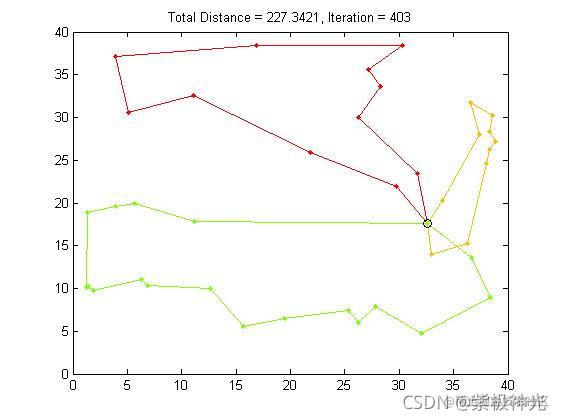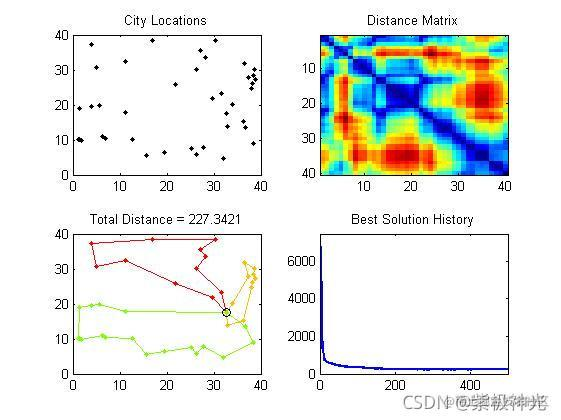## 五、matlab版本及参考文献

1 matlab版本 2014a

2 参考文献  包子阳,余继周,杨杉.智能优化算法及其MATLAB实例（第2版）[M].电子工业出版社，2016. 张岩,吴水根.MATLAB优化算法源代码[M].清华大学出版社，2017.